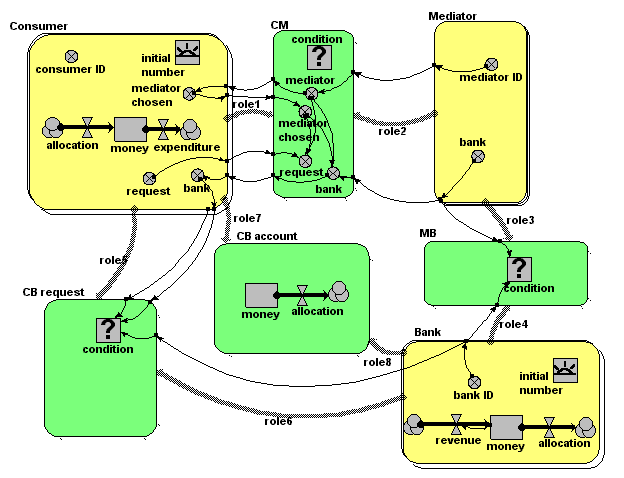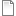# Banker/broker/consumer multi-agent model

ModelId:
dave1
SimileVersion:
3.1+

This model was developed in relation to the SLIE project (Sustainable Lifecycles for Information Ecosystems). That project is based on agent-based approaches: this model aims to explore the extent to which such models can be implemented in the Simile environment.Equations:

Equations in submodel Bank

creation: initial number = 2
compartment: money = 100000
flow: revenue = 0.1*money
flow: allocation = 0
variable: bank ID = index(1)

Equations in submodel Consumer
creation: initial number = 4
compartment: money = 0
flow: allocation = 0
flow: expenditure = 100
variable: consumer ID = index(1)
variable: request = (if rand_var(0,1)<0.2 then 1 else 0)
variable: mediator chosen = greatest({mediator})
variable: bank = sum({bank})

Equations in submodel Mediator
variable: mediator ID = index(1)
variable: bank = -1*floor(rand(1,2.9999))

Equations in submodel CM
condition: condition = (rand_const(0,1)<0.5)
variable: request = (if mediator==mediator_chosen then request else 0)
variable: mediator = mediator_id
variable: mediator chosen = mediator_chosen [in submodel Consumer]
variable: bank = (if mediator==mediator_chosen then bank else 0)

Equations in submodel MB
condition: condition = (bank==bank_id)

Equations in submodel CB request
condition: condition = (bank==bank_id&&request>0)

Equations in submodel CB account
compartment: money = 0

AttachmentSizedave1.sml122.15 KB